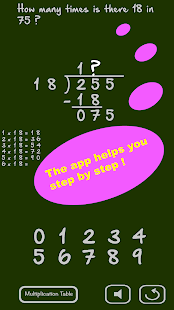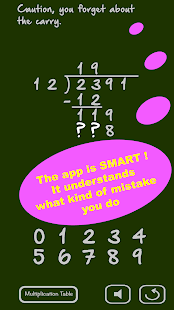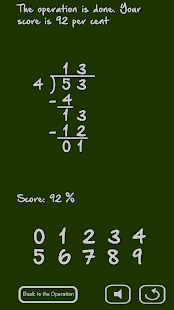### Math: Long Division v1.101 APK Add Free(No Ratings Yet)Loading...Description: The application will teach you how to make a long division. The application is smart: it tries to understand your mistakes and give you appropriate hints. The long division is a fundamental concept of mathematics. It includes the division of course, but also the multiplication, the subtraction and the multiplication tables, all mathematical operations. Mastering the long division means mastering all these techniques of mathematics. In learning mode, the application shows you how to do the long graduation step by step. Each step of the mathematical exercise is explained and detailed. You can select the numbers, divisor and dividend, or randomly select the application.

Supported Android
{4.1 and up}
Supported Android Version:-
Jelly Bean (4.1–4.3.1), KitKat (4.4–4.4.4), Lollipop (5.0–5.0.2) ,Marshmallow (6.0 – 6.0.1),Nougat (7.0–7.1.1), Oreo (8.0-8.1)

# Download Math: Long Division v1.101 APK Add Free Here

Long Division.ver.1.101.build.101-Signed.apk